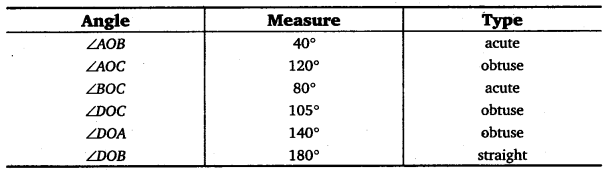# Class 6 Maths NCERT Solutions for Chapter 5 Understanding Elementary Shapes Ex – 5.4

## Understanding Elementary Shapes

Question 1.
What is the measure of

(i) a right angle?
(ii) a straight angle?

Solution:
(i) 1 right angle = 90°
(ii) 1 straight angle = 2 right angles = 2 x 90°=180°

Question 2.
Say True or False:

(a) The measure of an acute angle < 90°.
(b) The measure of an obtuse angle < 90°.
(c) The measure of a reflex angle > 180°.
(d) The measure of one complete revolution = 360°.
(e) If m∠A = 53° and m∠B = 35°, then m∠A > m∠B.

Solution:
(a) True
(b) False
(c) True
(d) True
(e) True

Question 3.
Write down the measures of

(a) some acute angles.
(b) some obtuse angles.
(give at least two examples of each).

Solution:
(a) Since the measure of an acute angle < 90°. So, two acute angles may be of 60° and 30°.
(b) Since the measure of an obtuse angle > 90°. So, two obtuse angles may be of 110° and 120°.

Question 4.
Measure the angles given below using the Protractor and write down the measure.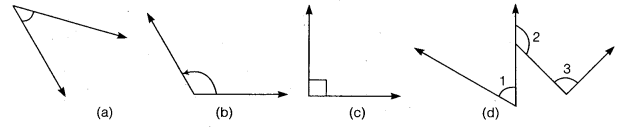Solution:
On measuring the given angles using protractor, we find that
(a) 45°
(b) 125°
(c) 90°
(d) ∠1 = 40°, ∠2 = 125° and ∠3 = 95°.

Question 5.
Which angle has a large measure ? First estimate and then measure.

Measure of Angle A =
Measure of Angle B =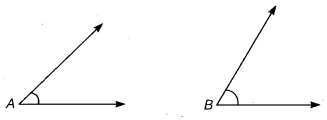Solution:
Observing the given angles, we find that
∠B > ∠A
On measuring, we find that
Measure of Angle A =40°
Measure of Angle B = 65°
∴ ∠B > ∠A.

Question 6.
From these two angles which has larger measure? Estimate and then confirm by measuring them.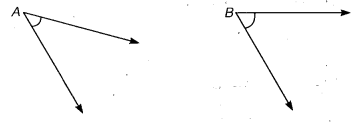Solution:
On observing, we find that
∠B > ∠A
On measuring, we find that
∠A = 45° and ∠B =60°
Thus, ∠B > ∠A.

Question 7.
Fill in the blanks with acute, obtuse, right or straight:

(a) An angle whose measure is less than that of a right angle is ……. .
(b) An angle whose measure is greater than that of a right angle is ……… .
(c) An angle whose measure is the sum of the measures of two right angles is ……. .
(d) When the sum of the measures of two angles is that of a right angle, then each one of them is …… .
(e) When the sum of the measures of two angles is that of a straight angle and if one of them is acute then the other should be …… .
Solution:
(a) acute
(b) obtuse
(c) straight
(d) acute
(e) obtuse

Question 8.
Find the measure of the angle shown in each figure. (First estimate with your eyes and then find the actual measure with a protractor).Solution:
On estimating without measuring, the measure of the angle shown in each figure is
(a) 45°
(b) 60°
(c) 125°
(d) 135°

On measuring with the protractor, we find their measures as under:
(a) 40°
(b) 65°
(c) 130°
(d) 135°

Question 9.
Find the angle measure between the hands of the clock in each figure:Solution:
The angle measure between the hands of the clock in the time shown is as under:
At 9.00 a.m. : 90°
At 1.00 p.m. : 30°
At 6.00 p.m. : 180°

Question 10.
Investigate

In the given figure, the angle measures 30°. Look at the same figure through a magnifying glass. Does the angle becomes larger? Does the size of the angle change?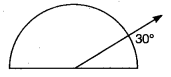Solution:
Looking through a magnifying glass does not make the angle larger and so the size of the angle does not change.

Question 11.
Measure and classify each angle: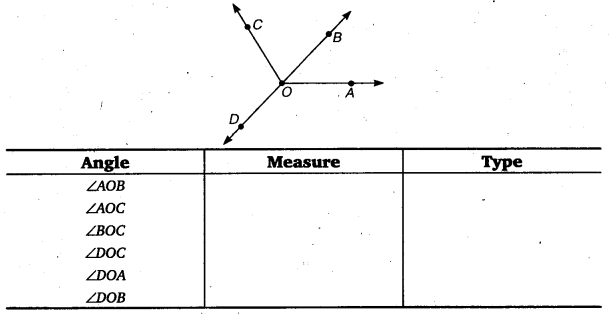Solution:
The measure of angle and its classification is recorded in the given table as under: